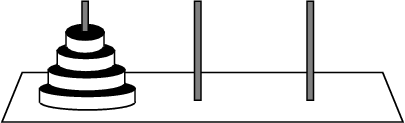### CSC 550: Introduction to Artificial Intelligence Fall 2004 HW3: State Space Search

This assignment involves solving state space problems and comparing the performance of the different search strategies discussed in class. MzScheme provides a utility function, time, that can be useful in profiling code. The time function, when given an expression as input, evaluates that expression and reports the number of milliseconds of CPU time taken for the evaluation. It even identifies the amount of CPU time taken by garbage collection, so that number can be subtracted out to determine the amount of time spent doing actual computation.

For example, the call (time (bfs '(0 0) '(2 0))) would report how long it took to solve the Jugs Puzzle using breadth-first search. Note, however, that the shortest time reportable by the time function is 10 ms. If a search succeeds in finding a solution in less than 10 ms, then it will register as no time at all. If this occurs, time multiple calls to the function and divide by the number of repetitions to get a more accurate timing. For example, the following call would time how long it takes for breadth-first search to solve the Jugs Puzzle five times.

(time (begin (bfs '(0 0) '(2 0)) (bfs '(0 0) '(2 0)) (bfs '(0 0) '(2 0)) (bfs '(0 0) '(2 0)) (bfs '(0 0) '(2 0))))

1. Consider each of the three state space problems discussed in class:

• travel.scm: 'Omaha --> 'LosAngeles
• jugs.scm: '(0 0) --> '(2 0)
• tiles.scm: '(1 2 3 8 6 space 7 5 4) --> '(1 2 3 8 space 4 7 6 5)

Using the time function and the implementations in search.scm, compare the performances of DFS, DFS-nocycles, BFS, BFS-nocycles, and DFS-deepening on each of these problems. That is, when the strategy produces an answer, report the execution time (not counting garbage collection). Comment on the apparent tradeoffs between the strategies.

2. Consider the problem of traversing a maze, from one opening (identified as the Start) to another opening (identified as the Exit). For example, the diagram on the left shows an 8x9 maze, where the Start is at the upper-left corner, the Exit is at the lower-right corner, and walls are shown as black rectangles. To the right is a Scheme list structure that represents this maze.

 S E
(define MAZE '("*********" "S *" "* ** * *" "* ** *" "* * *****" "* * *" "* * ** E" "*********"))

For maze traversals, a state space can be defined in which a state is defined by the coordinates of the person in the maze. For the above maze, we might identify the Start state as (1 0), designating the row at index 1 and the column at index 0. Likewise, the goal (Exit) state for this maze would be identified as (6 8).

The file maze.scm contains a Scheme definition of the state space for this problem. Using this implementation, compare the performances of the uninformed search strategies on this maze and comment on the apparent tradeoffs.

3. Similarly, compare the performances of the uninformed strategies on the following maze:

 S E

4. Consider the Towers of Hanoi Puzzle discussed in class.• Define a state space for this problem. In particular, determine how a state will be represented and define the GET-MOVES function to generate all states reachable from a given state. Your representation and implementation should be generic in that a puzzle with any number of discs could be solved using your code.
• Attempt to solve this problem using the various uninformed search control strategies provided, i.e. DFS, DFS-nocycles, BFS, BFS-nocycles, and DFS-deepening. Discuss the behaviors of each of these approaches. For example, if a strategy fails, explain why this would happen. Similarly, if one strategy outperforms another, explain why.